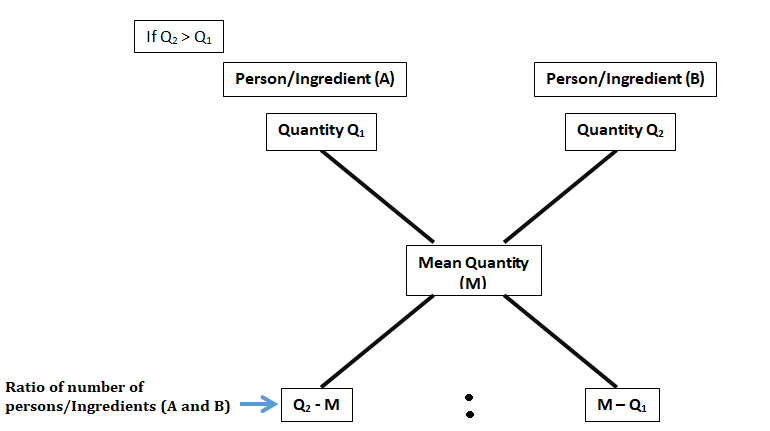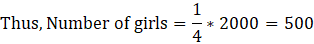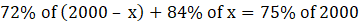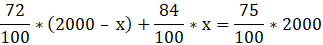Question of The Day19-12-2019

In an examination, out of 2000 students, 72% of boys and 84% of girls pass. If the total pass percentage was 75%. Find the total number of girls.

Correct Answer : b ) 500

Explanation :

Quick Approach:

Rule of Alligation: It states that when two similar types of quantities (like marks, ingredients, percentage, weight etc.) associated with two persons or ingredients are mixed together to obtain a mean combined quantity for those persons, thenThus, using the rule of alligationRatio of boys and girls = 3 : 1Hence, (B) is the correct answer.

Basic Approach:

Let the number of girls be x

Thus, number of boys will be 2000 – x

According to the question

Number of boys who pass = 72% of (2000 – x)

Number of girls who pass = 84% of x

Total number of students who pass = 75% of 2000

Thus,Solving for x we get,

x = 500

Hence, (b) is the correct answer.

Such type of question is asked in exams like SSC CGL, SSC MTS, SSC CPO, SSC CHSL, RRB JE, RRB NTPC, RRB GROUP D, RRB OFFICER SCALE-I, IBPS PO, IBPS SO, RRB Office Assistant, IBPS Clerk, RBI Assistant, IBPS RRB OFFICER SCALE 2&3, etc.

Read Daily Current Affairs, Banking Affairs and attempt free mock tests at PendulumEdu to prepare well for the government exams.0